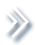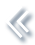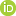####ArchivesReview Articles

## Semiparametric fractional imputation using empirical likelihood in survey sampling

Sixia Chen ,

University of Oklahoma, Oklahoma City, OK, USAJae kwang Kim

Iowa State University, Ames, IA, USAPages 69-81 | Received 07 Mar. 2017, Accepted 05 May. 2017, Published online: 01 Jun. 2021,
• Abstract
• References
• Citations

ABSTRACT

The empirical likelihood method is a powerful tool for incorporating moment conditions in statistical inference. We propose a novel application of the empirical likelihood for handling item non-response in survey sampling. The proposed method takes the form of fractional imputation but it does not require parametric model assumptions. Instead, only the first moment condition based on a regression model is assumed and the empirical likelihood method is applied to the observed residuals to get the fractional weights. The resulting semiparametric fractional imputation provides-consistent estimates for various parameters. Variance estimation is implemented using a jackknife method. Two limited simulation studies are presented to compare several imputation estimators.

## References

1. Chauvet, G., Deville, J. C., & Haziza, D. (2011). On balanced random imputation in surveys. Biometrika, 98, 459471.
2. Chen, J., & Sitter, R. (1999). A pseudo empirical likelihood approach to the effective use of auxiliary information in complex surveys. Statistica Sinica, 9, 385406.
3. Durrant, G. B., & Skinner, C. (2006). Using missing data methods to correct for measurement error in a distribution function. Survey Methodology, 32(1), 2536.
4. Fay, R. E. (1992). When are inferences from multiple imputation valid? In Proceedings of the Survey Research Methods Section of the American Statistical Association (Vol. 81, pp. 227232).
5. Fay, R. E. (1996). Alternative paradigms for the analysis of imputed survey data. Journal of the American Statistical Association, 91(434), 490498.
6. Fuller, W. A. (2009). Sampling statistics. Hoboken, NJ: Wiley.
7. Fuller, W. A., & Kim, J. K. (2005). Hot deck imputation for the response model. Survey Methodology, 31, 139149.
8. Haziza, D. (2009). Imputation and inference in the presence of missing data. In D. Pfeffermann & C. R. Rao (Eds.), Handbook of statistics. Sample surveys: Theory, methods and inference (Vol. 29, pp. 215246). Amsterdam: Elsevier BV.
9. Isaki, C. T. and Fuller, W. A. (1982). Survey design under the regression superpopulation model. Journal of the American Statistical Association, 77, 8996.
10. Kalton, G., & Kish, L. (1984). Some efficient random imputation methods. Communications in Statistics A, 13, 19191939.
11. Kim, J. K. (2011). Parametric fractional imputation for missing data analysis. Biometrika, 98, 119132.
12. Kim, J. K., Brick, J., Fuller, W. A., & Kalton, G. (2006). On the bias of the multiple-imputation variance estimator in survey sampling. Journal of Royal Statistical Society: Series B, 68(3), 509521.
13. Kim, J. K., & Fuller, W. A. (2004). Fractional hot deck imputation. Biometrika, 91(3), 559578.
14. Kim, J. K., Navarro, A., & Fuller, W. A. (2006). Replicate variance estimation after multi-phase stratified sampling. Journal of the American Statistical Association, 101, 312320.
15. Kim, J. K., & Shao, J. (2013). Statistical methods for handling incomplete data. London: Chapman and Hall/CRC.
16. Kim, J. K., & Yang, S. (2014). Fractional hot deck imputation for robust inference under item nonresponse in survey sampling. Survey Methodology, 40, 211230.
17. Kim, J. K., & Yu, C. L. (2011). A semi-parametric estimation of mean functionals with non-ignorable missing data. Journal of the American Statistical Association, 106, 157165.
18. Little, R. J. A., & Rubin, D. B. (2002). Statistical analysis with missing data (2nd ed.). Hoboken, NJ: Wiley.
19. Matloff, N. S. (1981). Use of regression functions for improved estimation of means. Biometrika, 68, 685689.
20. McCullagh, P., & Nelder, J. (1989). Generalized linear models. London: Chapman and Hall.
21. Meng, X. L. (1994). Multiple-imputation inferences with uncongenial sources of input. Statistical Science, 9, 538558.
22. Müller, U. U. (2009). Estimating linear functionals in nonlinear regression with response missing at random. Annals of Statistics, 98, 22452277.
23. Owen, A. B. (2001). Empirical likelihood. New York, NY: Chapman and Hall/CRC.
24. Qin, J. (1993). Empirical likelihood in biased sample problems. Annals of Statistics, 21(3), 11821196.
25. Qin, J., & Lawless, J. (1994). Empirical likelihood and general estimating equations. The Annals of Statistics, 22, 300325.
26. Rubin, D. B. (1987). Multiple imputation for nonresponse in surveys. New York: John Wiley & Sons.
27. Shao, J., & Steel, P. (1999). Variance estimation for survey data with composite imputation and nonnegligible sampling fractions. Journal of the American Statistical Association, 94, 254265.
28. Shao, J., & Tu, D. (1995). The jackknife and bootstrap. New York: Springer-Verlag.
29. Vardi, Y. (1985). Empirical distributions in selection bias models. Annals of Statistics, 13, 178203.
30. Van der Vaart, A. W. (1998). Asymptotic statistics. New York: Cambridge University Press.
31. Vís̆ek, J. A. (1979). Asymptotic distribution of simple estimate for rejective, Sampford and successive sampling. In J. Jurecková (Ed.), Contributions to statistics: Jaroslav hj́ek memorial volume (pp. 263275). Dordrecht: Academia, Prague & D. Reidel.
32. Wang, D., & Chen, S. X. (2009). Empirical likelihood for estimating equations with missing values. The Annals of Statistics, 37, 490517.33. Wang, Q., & Rao, J. N. K. (2002). Empirical likelihood-based inference under imputation for missing response data. The Annals of Statistics, 30, 896924.34. Wang, N., & Robins, J. M. (1998). Large-sample theory for parametric multiple imputation procedures. Biometrika, 85(4), 935948.
35. Wang, J. Q., & Opsomer, J. D. (2011). On asymptotic normality and variance estimation for nondifferentiable survey estimators. Biometrika, 98, 91106.
36. Wolter, K. M. (2007). Introduction to variance estimation. New York, NY: Wiley.
37. Wu, C., & Rao, J. N. K. (2006). Pseudo empirical likelihood ratio confidence intervals for complex surveys. The Canadian Journal of Statistics, 34, 359375.
38. Yang, S., & Kim, J. K. (2016). A note on multiple imputation for general-purpose estimation. Biometrika, 103, 244251.

Niansheng Tang, Yuanyuan Ju. (2018) Statistical inference for nonignorable missing-data problems: a selective review. Statistical Theory and Related Fields 2:2, pages 105-133.